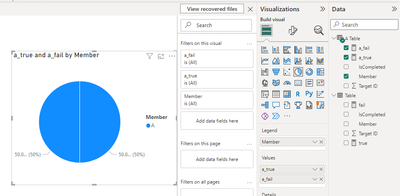cancel
Showing results for
Did you mean:Helper IV

## Multiple (Dynamic List) of Pie Or Gauge or donut chart

I want to have a dynamic number of Pie Or Gauge or donut chart.

Columns are :  Member | Target ID | IsCompleted (True/False).

So: Each pie should be for each member and it should show: Count Of Target ID, lets say, its 10 for Member A. and then let's say 5 of then are : IsCompleted: True; then Chart should show 50% filled.  This way for each member a seperate Pie.

All chart should be in a single row. Just like the way, Small Multiple works and there you can adjust grid etc.

I see so far no such options. I tried with R visual. But the kind of function and libraries I used, require at least R 4.2.2 where as Power BI service I guess offer only R 3.4.4

Has anyone came across such scenario and found any work around?

1 ACCEPTED SOLUTIONCommunity Support

Hi @RKM ,

According to your description, here are my steps you can follow as a solution.

(1) This is my test data.(2) We can create a table and measures.

``A Table= FILTER('Table','Table'[Member]="A")``
``````true =
var _a =COUNTROWS(FILTER(ALL('Table'),'Table'[Member]=MAX('Table'[Member])))
var _b=COUNTROWS(FILTER(ALL('Table'),'Table'[Member]=MAX('Table'[Member]) && 'Table'[IsCompleted]="TRUE"))
return DIVIDE(_b,_a,0)``````
``fail = 1-'Table'[true]``
``````a_true =
var _a =COUNTROWS(FILTER(ALL('A Table'),'A Table'[Member]=MAX('A Table'[Member])))
var _b=COUNTROWS(FILTER(ALL('A Table'),'A Table'[Member]=MAX('A Table'[Member]) && 'A Table'[IsCompleted]="TRUE"))
return DIVIDE(_b,_a,0)``````
``a_fail = 1-'A Table'[true]``

(3) Then the result is as follows.If the above one can't help you get the desired result, please provide some sample data in your tables (exclude sensitive data) with Text format and your expected result with backend logic and special examples. It is better if you can share a simplified pbix file. Thank you.

Best Regards,

Neeko Tang

If this post  helps, then please consider Accept it as the solution  to help the other members find it more quickly.Community Support

Hi @RKM ,

According to your description, here are my steps you can follow as a solution.

(1) This is my test data.(2) We can create a table and measures.

``A Table= FILTER('Table','Table'[Member]="A")``
``````true =
var _a =COUNTROWS(FILTER(ALL('Table'),'Table'[Member]=MAX('Table'[Member])))
var _b=COUNTROWS(FILTER(ALL('Table'),'Table'[Member]=MAX('Table'[Member]) && 'Table'[IsCompleted]="TRUE"))
return DIVIDE(_b,_a,0)``````
``fail = 1-'Table'[true]``
``````a_true =
var _a =COUNTROWS(FILTER(ALL('A Table'),'A Table'[Member]=MAX('A Table'[Member])))
var _b=COUNTROWS(FILTER(ALL('A Table'),'A Table'[Member]=MAX('A Table'[Member]) && 'A Table'[IsCompleted]="TRUE"))
return DIVIDE(_b,_a,0)``````
``a_fail = 1-'A Table'[true]``

(3) Then the result is as follows.If the above one can't help you get the desired result, please provide some sample data in your tables (exclude sensitive data) with Text format and your expected result with backend logic and special examples. It is better if you can share a simplified pbix file. Thank you.

Best Regards,

Neeko Tang

If this post  helps, then please consider Accept it as the solution  to help the other members find it more quickly.Announcements#### Exclusive opportunity for Women!

Join us for a free, hands-on Microsoft workshop led by women trainers for women where you will learn how to build a Dashboard in a Day!#### Power Platform Conference-Power BI and Fabric Sessions

Join us Oct 1 - 6 in Las Vegas for the Microsoft Power Platform Conference.Top Solution Authors
Top Kudoed Authors
Users online (2,956)